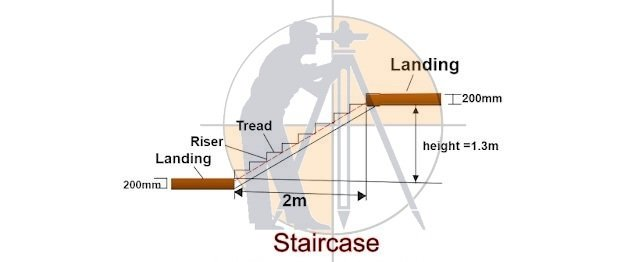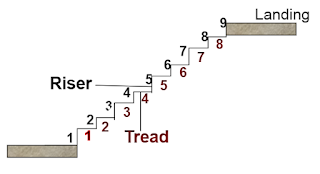Breaking News
Home / Civil Engineering / How To Calculate The Numbers Of tread And Riser In Staircase

# How To Calculate The Numbers Of tread And Riser In Staircase

## How To Calculate The Numbers Of tread And Riser In Staircase

Let us calculate the number of riser & tread for the below-given drawing.### Given data:

Landing slab thickness = 200mm.
Top to bottom surface landing height = 1.3m. = 1300mm.
Inner span between the landings = 2m. = 2000mm.
First, let us assume the riser depth as =160mm.So, the number of risers= landing height ÷ riser height.Top to top landing height= Top to bottom height of landing + landing thickness= 1300mm + 200mm.= 1500mm.The no. of risers

### Some useful tips on volume calculation of concrete staircase

As the risers should be the whole number, let us provide 9 nos. of risers & we will divide the landing height with the number of rises to get the corrected riser depth.

### The actual height of the riser

= landing height ÷ no. of risers

= 1500mm ÷ 9nos.

### Now, the number of treads

= number of riser – 1From the above drawing, you can observe that the total no. of treads is always less by one from the given no. of risers.

### = 8 nos.

= horizontal clear span between landings ÷ the no. of treads.

### THANKS.